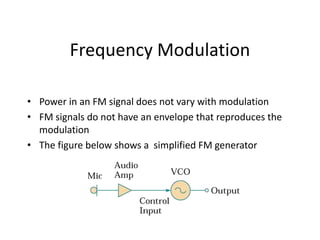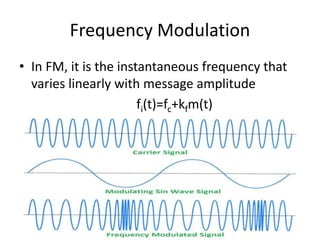Ce diaporama a bien été signalé.
Le téléchargement de votre SlideShare est en cours. ×

# Angle modulationChargement dans…3
×

1 sur 22
1 sur 22

# Angle modulation

### Angle modulation

1. 1. Angle Modulation Frequency Modulation
2. 2. Frequency Modulation • Power in an FM signal does not vary with modulation • FM signals do not have an envelope that reproduces the modulation • The figure below shows a simplified FM generator
3. 3. 3 Frequency Modulation • In FM, it is the instantaneous frequency that varies linearly with message amplitude fi(t)=fc+kfm(t)
4. 4. Frequency Modulation • The modulating signal changes the freq. fc of the carrier signal • The bandwidth for FM is high • It is approx. 10x the signal frequency
5. 5. Frequency Deviation • Frequency deviation of the carrier is proportional to the amplitude of the modulating signal as illustrated
6. 6. Frequency Modulation Index • Another term common to FM is the modulation index, as determined by the formula: m f f m  
7. 7. 7 FM for Tone Signals • Consider a sinusoidal message • The instantaneous frequency corresponding to its FM version is  tfAtm mm 2cos)(      tfAkf tmkftf mmf frequencyresting c fci 2cos )(  
8. 8. 8 Frequency Deviation • Inst. frequency has upper and lower bounds given by     fff fff then Akdeviationfrequencyf where tffftf ci ci mf mci     min max 2cos 
9. 9. 5.9 Frequency modulation
10. 10. FM Modulation using VCO • VCO free running frequency = Carrier Frequency • VCO Frequency Range is no smaller than Bandwidth
11. 11. Block Diagram DC Bias Vcc/2  VCO PAInput
12. 12. FM Modulation using VCO  tmcfci        cdttmctt ft      dttmctAS fccPM cos Vin fout fC - Gain of VCO c - Free Running Frequency of VCO Corresponding DC bias 
13. 13. Bandwidth of FM is infinite • As in the case of AM, this time domain representation of the FM signal can be converted to an equivalent frequency-domain expression that includes the carrier and sidebands. Because the mathematics required for this conversion are quite complex, we will only consider the result: • The Jn(x) functions are known as Bessel Functions of the First Kind. Graphs of Jn(x) look like slowly decreasing sine and cosine functions. The Jn(x) functions are a closely related family of functions in the same way that sin(nx) and cos(nx) for a family of similar functions. The zeroth order Bessel function, J0(m) determines the amplitude of the carrier. The nth Bessel function Jn(m) determines the amplitude of the nth pair of sidebands. There are two important concepts contained in the expression shown above The amplitude of the carrier depends on m. the modulation index. This is quite different from AM, where the amplitude of the carrier was independent of the value of m There are an infinite number of sidebands. Thus the theoretical bandwidth of FM is infinite.
14. 14. An infinite bandwidth signal would be very difficult to transmit. Fortunately, the higher order sidebands in FM have extremely low amplitude and may be ignored. For example: if the modulation index is 5, only the first 7 sidebands are significant in value.
15. 15. Narrowband and Wideband FM • There are no theoretical limits to the modulation index or the frequency deviation of an FM signal • The limits are a practical compromise between signal-to- noise ratio and bandwidth • Government regulations limit the bandwidth of FM transmissions in terms of maximum frequency deviation and the maximum modulation frequency
16. 16. Narrow- and Wideband Signals • Narrowband FM (NBFM) is used for voice transmissions • Wideband FM (WBFM) is used for most other transmissions • Strict definition of the term narrowband FM refers to a signal with mf of less than 0.5
17. 17. 17 FM signal demodulation • It is more resistant to noise than an AM signal. • filtering and Limiting the transmitted signal. • Differentiation to obtain the phase information in the modulated signal. • There are four ways to implement differentiation:  Phase-Locked Loop  Zero-Crossing Detection  FM-to-AM Conversion  Phase-Shift or Quadrature Detection
18. 18. 18 Phase-Locked Loop (PLL) The PLL consists of three basic components: A. Phase detector (PD) B. Low-pass filter (LPF) C. Voltage controlled oscillator (VCO) Sout ( t )Sf ( t ) Sphase( t ) Voltage Controlled Oscillator (VCO) SVCO ( t ) = AVCO ·sin [ 0 t +  0( t )] Sf ( t ) = Af ·cos [ c t +  ( t )] SVCO ( t ) Phase Detector Low-pass filter
19. 19. Phase-Locked Loop (PLL) • A phase detector compares two input signals and produces an error signal which is proportional to their phase difference. • If the output phase drifts, the error signal will increase, driving the VCO phase in the opposite direction so as to reduce the error. Thus the output phase is locked to the phase at the other input. This input is called the reference. • The output is fed through an optional divider back to the input of the system, producing a negative feedback loop.
20. 20. Phase-Locked Loop (PLL) • Analog phase locked loops are generally built with an analog phase detector, low pass filter and VCO placed in a negative feedback configuration. A digital phase locked loop uses a digital phase detector; it may also have a divider in the feedback path or in the reference path, or both, in order to make the PLL's output signal frequency a rational multiple of the reference frequency.
21. 21. 21 Demodulation by Zero Crossing Detection • Zero crossing detector • Positive voltage. • Negative voltage. • Pulse generator. • low-pass filter. • The advantage of zero crossing detection (and FM-to-AM conversion) is that no source of the carrier frequency is required to demodulate the signal. A digital signal can easily be recovered from a FM signal in this manner. • Decoding an analog signal may be difficult by this method, since the signal at the low-pass filter output does not closely resemble the baseband signal.
22. 22. Zero crossing detector Hard limiter Zero Crossing detector Multi- vibrator Averaging circuit FM Output FM input Hard limiter ZC detector multiV more frequent ZC’s means higher inst freq in turn means Larger message amplitudes Averaging circuit